# Applied 40S April 21, 2009

21 de Apr de 2009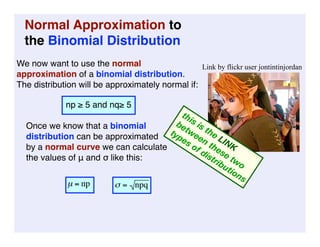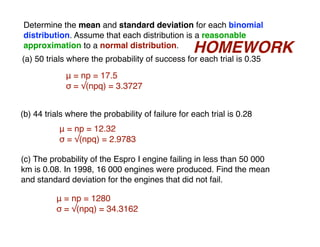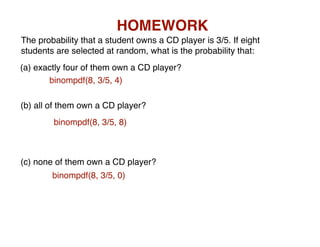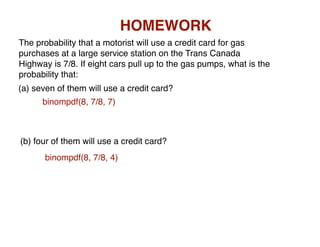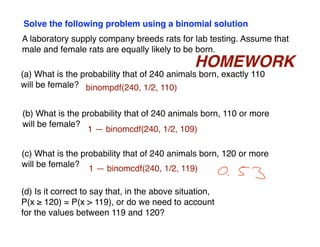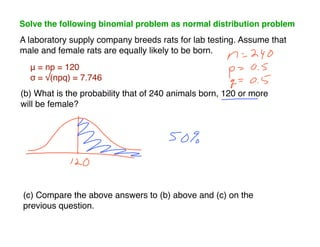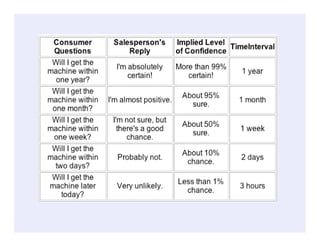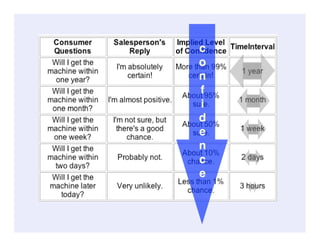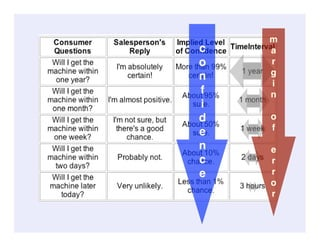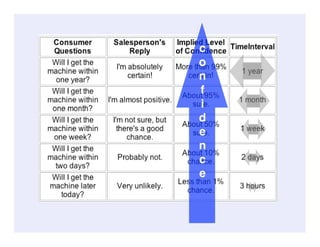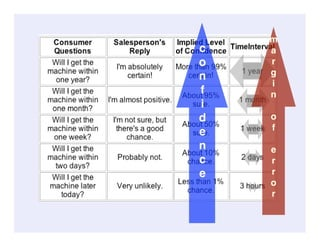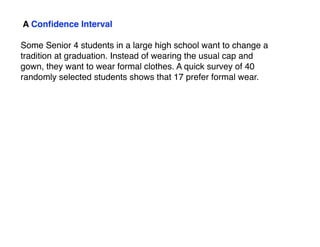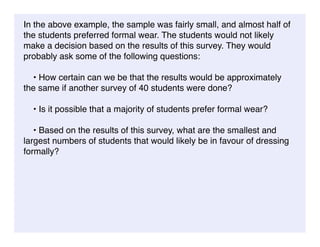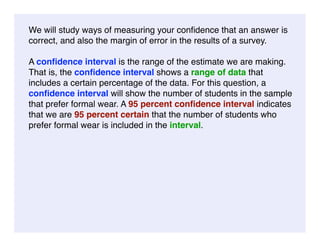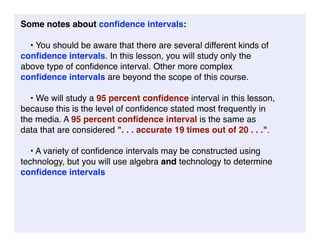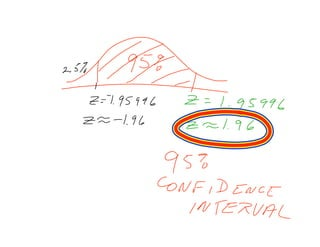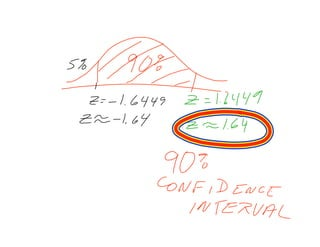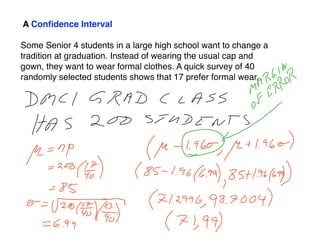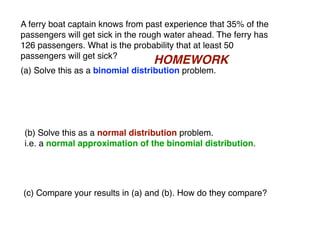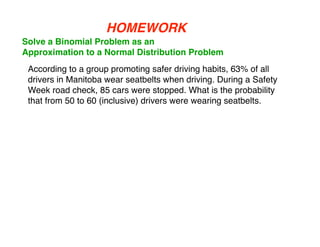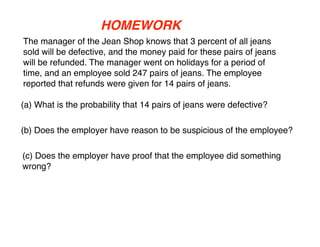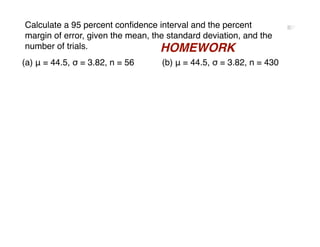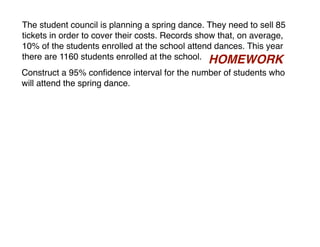1 de 25

### Applied 40S April 21, 2009

• 1. Required: A High Level of Conﬁdence and a Small Margin of Error The Formation 1 by ﬂickr user satosphere
• 2. Normal Approximation to the Binomial Distribution We now want to use the normal Link by flickr user jontintinjordan approximation of a binomial distribution. The distribution will be approximately normal if: np ≥ 5 and nq≥ 5 th be is is Once we know that a binomial typ twe the es en LIN distribution can be approximated of the K by a normal curve we can calculate dis se tri tw the values of μ and σ like this: bu o tio ns
• 3. Determine the mean and standard deviation for each binomial distribution. Assume that each distribution is a reasonable HOMEWORK approximation to a normal distribution. (a) 50 trials where the probability of success for each trial is 0.35 μ = np = 17.5 σ = √(npq) = 3.3727 (b) 44 trials where the probability of failure for each trial is 0.28 μ = np = 12.32 σ = √(npq) = 2.9783 (c) The probability of the Espro I engine failing in less than 50 000 km is 0.08. In 1998, 16 000 engines were produced. Find the mean and standard deviation for the engines that did not fail. μ = np = 1280 σ = √(npq) = 34.3162
• 4. HOMEWORK The probability that a student owns a CD player is 3/5. If eight students are selected at random, what is the probability that: (a) exactly four of them own a CD player? binompdf(8, 3/5, 4) (b) all of them own a CD player? binompdf(8, 3/5, 8) (c) none of them own a CD player? binompdf(8, 3/5, 0)
• 5. HOMEWORK The probability that a motorist will use a credit card for gas purchases at a large service station on the Trans Canada Highway is 7/8. If eight cars pull up to the gas pumps, what is the probability that: (a) seven of them will use a credit card? binompdf(8, 7/8, 7) (b) four of them will use a credit card? binompdf(8, 7/8, 4)
• 6. Solve the following problem using a binomial solution A laboratory supply company breeds rats for lab testing. Assume that male and female rats are equally likely to be born. HOMEWORK (a) What is the probability that of 240 animals born, exactly 110 will be female? binompdf(240, 1/2, 110) (b) What is the probability that of 240 animals born, 110 or more will be female? 1 — binomcdf(240, 1/2, 109) (c) What is the probability that of 240 animals born, 120 or more will be female? 1 — binomcdf(240, 1/2, 119) (d) Is it correct to say that, in the above situation, P(x ≥ 120) = P(x > 119), or do we need to account for the values between 119 and 120?
• 7. Solve the following binomial problem as normal distribution problem A laboratory supply company breeds rats for lab testing. Assume that male and female rats are equally likely to be born. μ = np = 120 σ = √(npq) = 7.746 (b) What is the probability that of 240 animals born, 120 or more will be female? (c) Compare the above answers to (b) above and (c) on the previous question.
• 8. CONFIDENCE INTERVALS Mike wants to buy a new electronic machine that can modify CDs and DVDs. The machine has been advertised on TV, is apparently available in the U.S. and parts of Western Europe, but is currently not available in the local stores. The shop manager has ordered some of the machines, but has received no response from the supplier. Mike needs the machine for his business, and so he asks the salesperson when he will get the machine if he orders it now. The table shows a record of the conversation between Mike and the salesperson.
• 11. m c a o r g n i f n i o d f e n e c r r e o r
• 13. m c a o r g n i f n i o d f e n e c r r e o r
• 14. A Conﬁdence Interval Some Senior 4 students in a large high school want to change a tradition at graduation. Instead of wearing the usual cap and gown, they want to wear formal clothes. A quick survey of 40 randomly selected students shows that 17 prefer formal wear.
• 15. In the above example, the sample was fairly small, and almost half of the students preferred formal wear. The students would not likely make a decision based on the results of this survey. They would probably ask some of the following questions: • How certain can we be that the results would be approximately the same if another survey of 40 students were done? • Is it possible that a majority of students prefer formal wear? • Based on the results of this survey, what are the smallest and largest numbers of students that would likely be in favour of dressing formally?
• 16. We will study ways of measuring your conﬁdence that an answer is correct, and also the margin of error in the results of a survey. A conﬁdence interval is the range of the estimate we are making. That is, the conﬁdence interval shows a range of data that includes a certain percentage of the data. For this question, a conﬁdence interval will show the number of students in the sample that prefer formal wear. A 95 percent conﬁdence interval indicates that we are 95 percent certain that the number of students who prefer formal wear is included in the interval.
• 17. Some notes about conﬁdence intervals: • You should be aware that there are several different kinds of conﬁdence intervals. In this lesson, you will study only the above type of conﬁdence interval. Other more complex conﬁdence intervals are beyond the scope of this course. • We will study a 95 percent conﬁdence interval in this lesson, because this is the level of conﬁdence stated most frequently in the media. A 95 percent conﬁdence interval is the same as data that are considered quot;. . . accurate 19 times out of 20 . . .quot;. • A variety of conﬁdence intervals may be constructed using technology, but you will use algebra and technology to determine conﬁdence intervals
• 20. A Conﬁdence Interval Some Senior 4 students in a large high school want to change a tradition at graduation. Instead of wearing the usual cap and gown, they want to wear formal clothes. A quick survey of 40 randomly selected students shows that 17 prefer formal wear.
• 21. A ferry boat captain knows from past experience that 35% of the passengers will get sick in the rough water ahead. The ferry has 126 passengers. What is the probability that at least 50 passengers will get sick? HOMEWORK (a) Solve this as a binomial distribution problem. (b) Solve this as a normal distribution problem. i.e. a normal approximation of the binomial distribution. (c) Compare your results in (a) and (b). How do they compare?
• 22. HOMEWORK Solve a Binomial Problem as an Approximation to a Normal Distribution Problem According to a group promoting safer driving habits, 63% of all drivers in Manitoba wear seatbelts when driving. During a Safety Week road check, 85 cars were stopped. What is the probability that from 50 to 60 (inclusive) drivers were wearing seatbelts.
• 23. HOMEWORK The manager of the Jean Shop knows that 3 percent of all jeans sold will be defective, and the money paid for these pairs of jeans will be refunded. The manager went on holidays for a period of time, and an employee sold 247 pairs of jeans. The employee reported that refunds were given for 14 pairs of jeans. (a) What is the probability that 14 pairs of jeans were defective? (b) Does the employer have reason to be suspicious of the employee? (c) Does the employer have proof that the employee did something wrong?
• 24. Calculate a 95 percent conﬁdence interval and the percent (a) 95% conﬁdence interval =µ ± 1.96σ = 44.5 ± 1.96(3.82) = 44.5 ± 7.49 95% conﬁdence interval = (37.01, 51.99) Margin of error = ± 1.96σ = 7.49 % margin of error = ±13.38% (b) 95% conﬁdence interval =µ ± 1.96σ = 44.5 ± 1.96(3.82) = 44.5 ± 7.49 95% conﬁdence interval = (37.01, 51.99) Margin of error = ± 1.96σ = 7.49 % margin of error = ±1.74% (c) 95% conﬁdence interval = 540 ± 16.66 = (523.34, 556.66) Margin of error = ± 1.96σ = ± 16.66 % margin of error = ± 8.33% margin of error, given the mean, the standard deviation, and the HOMEWORK number of trials. (b) μ = 44.5, σ = 3.82, n = 430 (a) μ = 44.5, σ = 3.82, n = 56
• 25. The student council is planning a spring dance. They need to sell 85 tickets in order to cover their costs. Records show that, on average, 10% of the students enrolled at the school attend dances. This year there are 1160 students enrolled at the school. HOMEWORK Construct a 95% conﬁdence interval for the number of students who will attend the spring dance.